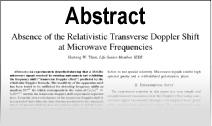Enter the content which will be displayed in sticky bar

AbstractSpace and Time Are Better Than Space-Time (Parts I & II)

Year: 2000
Keywords: Space,Time, Space-Time
Part 1. Transformations of space and time between inertial systems have been constructed by assuming: (1) Invariance of the two way velocity of light; (2) Retardation by the usual velocity dependent factor for clocks moving with respect to an isotropical system. The so obtained transformations contain a free parameter e1. Different values of e1 correspond to different theories of space-time phenomena. The Theory of Special Relativity (TSR) is recovered for a particular choice of e1. Many experiments are insensitive to the choice of e1: Michelson type, aberration, occultations of Jupiter satellites, radar ranging of planets and so on. There is however at least an exception (see part II).

Part 2. A physical quantity rho exists for which the TSR predicts rho: 1 relative to all inertial frames. Under extremely general conditions we show that rho={c+v)/(c-v) for all rotating disks having the same peripheral velocity v. This rho must hold in any small region near the disk rim and arbitrarily small acceleration a. Therefore the TSR gives rise to a discontinuity which is unacceptable because all empirical knowledge about inertial systems is obtained in frames with nonzero a, e.g. because of the Earth,s rotation. Elimination of the discontinuity is possible using the set of equivalent theories. The clock synchronization ambiguity in inertial systems is then solved: only e1=0 (corresponding to absolute simultaneity) gives rho-{c+v)/(c-v) when a goes to zero. Noninvariant values of the one way velocity of light are thus obtained and a new theoretical framework emerges in which space and time are not mixed and the usual relativistic paradoxes are absent.